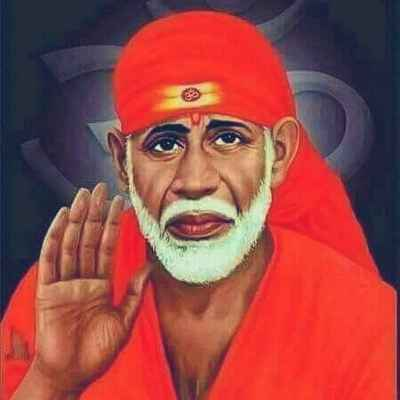Forums

# Proportions

If A:B=3:2 AND B:C=3:5 then A:B:C is Q no.13

AttachmentsRitesh Pandey

CA Foundation

680

16-May-20

66

Did you try and could not get the answer?Lavanya Chitturi

CA Inter

895

16-May-20

Mam i got my answer nowRitesh Pandey

CA Foundation

680

16-May-20

9:6:10

Attachments

3c30255c-8d7c-495f-9d38-c2894e19c3a0.jpgRitesh Pandey

CA Foundation

680

16-May-20

Is there any sequence or formula to find out this answer Or there simply compare the options with ratio to get it. Plz reply...Pranav Connecting...

CA Inter

4K+

16-May-20

Pranav Connecting...

Is there any sequence or formula to find out this answer Or there simply compare the options with ratio to get it. Plz reply...

In A and b and in B and C B should be Samesai t

CA Foundation

305

16-May-20

Yea..plz complete the precedurePranav Connecting...

CA Inter

4K+

16-May-20

Suppose A:B =3:5 and B:C =2:6 Then so to get Same number I mean similar number multiply 5 with 2 and 2 with 5 then A:B=3:10 B:C=10:6 therefore A:B:C= 3:10:6 same principle apply's for above problemsai t

CA Foundation

305

16-May-20

sai t

Suppose A:B =3:5 and B:C =2:6 Then so to get Same number I mean similar number multiply 5 with 2 and 2 with 5 then A:B=3:10 B:C=10:6 therefore A:B:C= 3:10:6 same principle apply's for above problem

Yea., I got it.. answer 6:10:30.. ThanksPranav Connecting...

CA Inter

4K+

17-May-20

Sales